edraw-max-horizontal
Guide
What's New
TRY IT FREE

Examples for Algorithm Flowcharts

> Flowchart Examples > Examples for Algorithm Flowcharts
Algorithms and flowcharts are two different ways of presenting the process of solving a problem. Algorithms consist of a set of steps for solving a particular problem, while in flowcharts, those steps are usually displayed in shapes and process boxes with arrows. So flowcharts can be used for presenting algorithms. This page will introduce some examples of algorithm flowcharts.

Flowcharts are diagrams that visually present the process of solving problems. They are drawn according to steps described in the algorithms. On this page you will find some flowchart examples explaining the algorithms.

Rules of Drawing Flowcharts for Algorithms

There are some basic shapes and boxes included in flowcharts that are used in the structure of explaining steps of algorithms. Knowing how to use them while drawing flowcharts is crucial. Here are some rules that should be known:

1. All boxes of flowcharts are connected with arrows to show the logical connection between them,
2. Flowcharts will flow from top to bottom,
3. All flowcharts start with a Start Box and end with a Terminal Box,

Examples of Flowcharts for Algorithms

These examples will help you get a better understanding of flowchart techniques.

Example 1: Calculate the Interest of a Bank Deposit

Algorithm:

• Step 1: Read amount,
• Step 2: Read years,
• Step 3: Read rate,
• Step 4: Calculate the interest with formula "Interest=Amount*Years*Rate/100
• Step 5: Print interest,

Flowchart: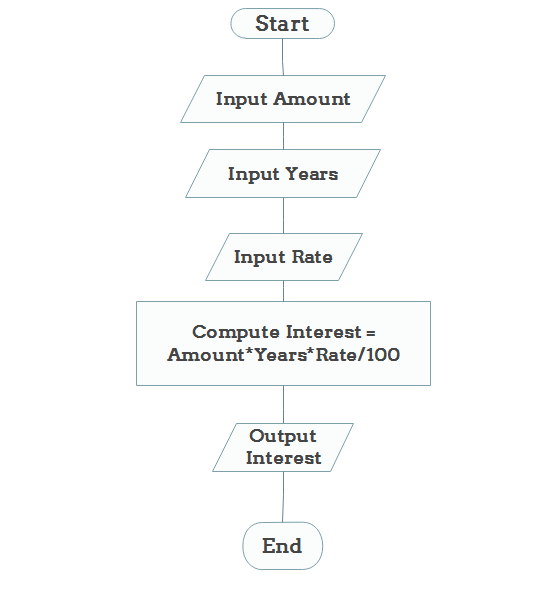Example 2: Determine and Output Whether Number N is Even or Odd

Algorithm:

• Step 1: Read number N,
• Step 2: Set remainder as N modulo 2,
• Step 3: If remainder is equal to 0 then number N is even, else number N is odd,
• Step 4: Print output.

Flowchart: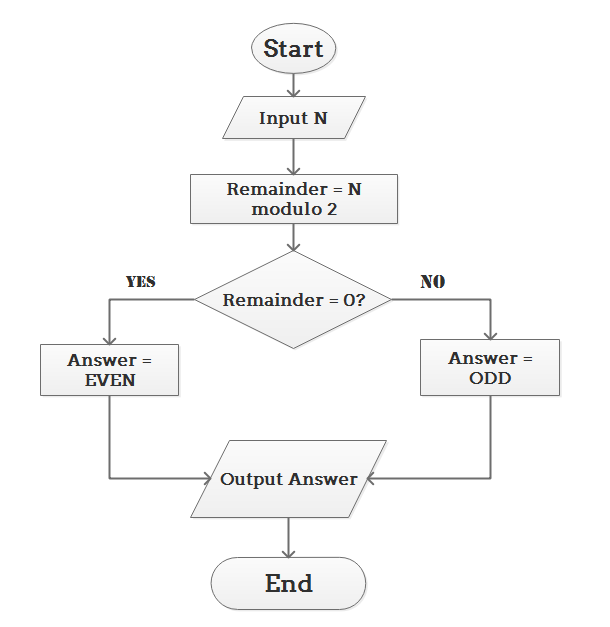Example 3: Determine Whether a Temperature is Below or Above the Freezing Point

Algorithm:

• Step 1: Input temperature,
• Step 2: If it is less than 32, then print "below freezing point", otherwise print "above freezing point"

Flowchart: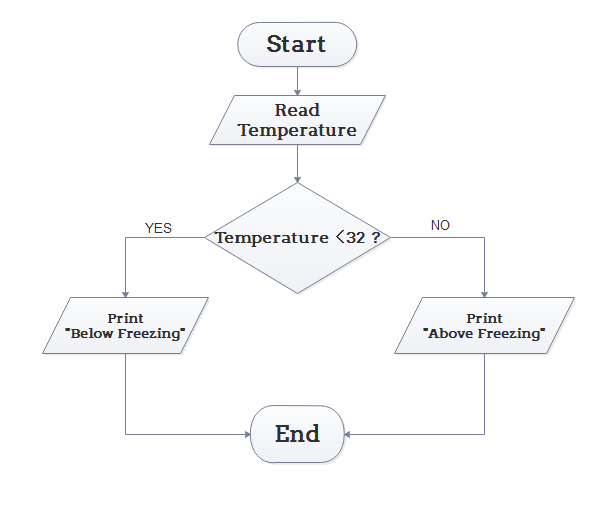Example 4: Determine Whether A Student Passed the Exam or Not:

Algorithm:

• Step 1: Input grades of 4 courses M1, M2, M3 and M4,
• Step 2: Calculate the average grade with formula "Grade=(M1+M2+M3+M4)/4"
• Step 3: If the average grade is less than 60, print "FAIL", else print "PASS".

Flowchart: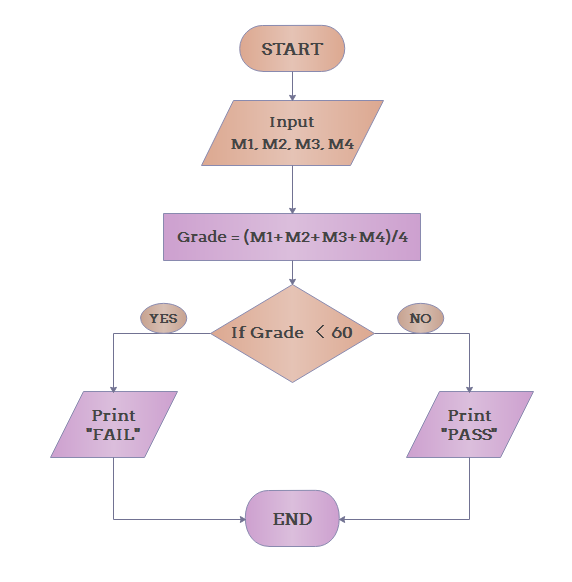NOTES

1. Adding Shapes in Flowcharts:

While drawing flowcharts with Edraw flowchart maker, shapes can be simply dragged and dropped beside the box at any direction (right, left, up or below) with the help of mouse, and they will be connected by arrows automatically.

As shown in this picture: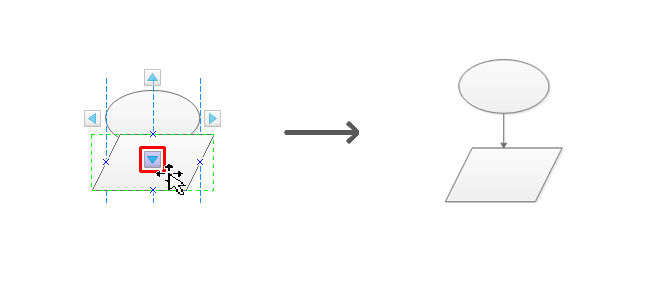Click the icon on the side of the box, shapes will be added automatically at any direction needed. Types of shapes can be modified by clicking on the icon at the top right corner and choosing the shape needed.

As shown in the picture below: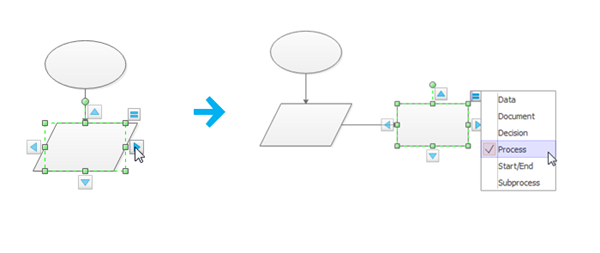2. Themes and Colors:

Under the "Layout" menu, the two buttons shown in the picture can help with the theme and the color matching of your flowcharts. If your time is limited or simply cannot come up with the right color matching, why not just pick one from the various color combinations prepared by our professional designers?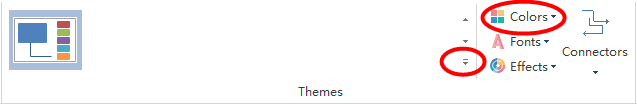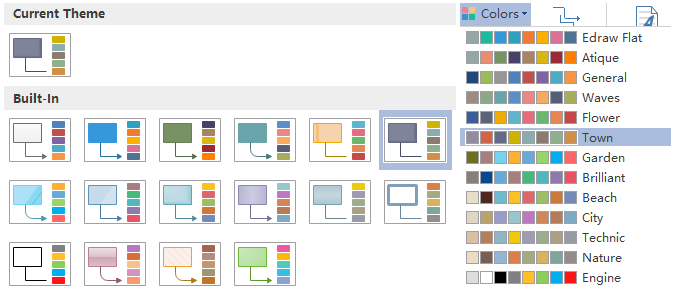Flowchart is a better way of presenting the steps of solving a problem and the concerned logical system that described in an algorithm. It provides a more effective analysis of the problem.